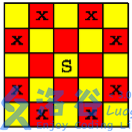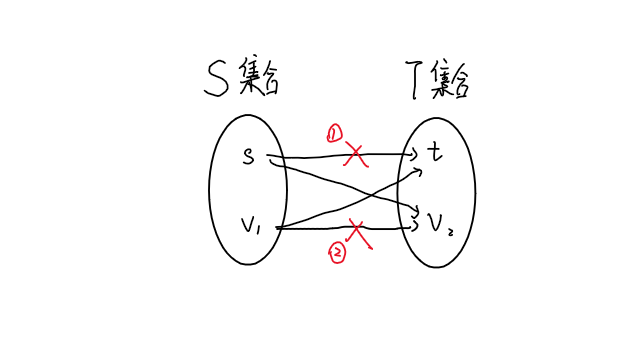# 0.板子

struct edge {
int cap,flow;
}e[N];

vector <pii> G[N];

int n,m,s,t,tot = -1,dis[N],cur[N];
bool vis[N];

bool BFS() {
memset(dis,0,sizeof dis);
memset(vis,0,sizeof vis);
queue <int> q;
q.push(s);dis[s] = 0;vis[s] = 1;
while (!q.empty()) {
int x = q.front();q.pop();
for (auto ed : G[x]) {
int y = ed.first,num = ed.second;
if (!vis[y] && e[num].cap > e[num].flow) {
vis[y] = 1;
dis[y] = dis[x] + 1;
q.push(y);
}
}
}
return vis[t];
}

int DFS(int x,int res) {
if (x == t || res == 0) return res;
int now = 0;
for (int& i = cur[x];i < G[x].size();++i) {
pii ed = G[x][i];
int y = ed.first,num = ed.second;
if (dis[x] + 1 == dis[y]) {
int f = DFS(y,min(res,e[num].cap - e[num].flow));
if (f > 0) {
e[num].flow += f;
e[num ^ 1].flow -= f;
now += f;
res -= f;
if (!res) break;
}
}
}
return now;
}

int maxflow() {
int ans = 0;
while (BFS()) {
memset(cur,0,sizeof cur);
ans += DFS(s,0x3f3f3f3f);
}
return ans;
}

void addedge(int x,int y,int w) {
G[x].push_back(mp(y,++tot));
e[tot].cap = w;e[tot].flow = 0;
G[y].push_back(mp(x,++tot));
e[tot].cap = 0;e[tot].flow = 0;
}

vector <pii> G[N];

struct edge {
int from,cap,flow,cost;
}e[N];

int tot = -1,s,t;

void addedge(int u,int v,int w,int co) {
e[++tot].cap = w;e[tot].cost = co;e[tot].flow = 0;e[tot].from = u;
G[u].push_back(mp(v,tot));
e[++tot].cap = 0;e[tot].cost = -co;e[tot].flow = 0;e[tot].from = v;
G[v].push_back(mp(u,tot));
}

int dis[N],inq[N],p[N],aaa[N],n,m;

bool Ford(int &flow,int &cost) {
memset(dis,0x3f,sizeof dis);
memset(inq,0,sizeof inq);
queue <int> q;
dis[s] = 0;
inq[s] = 1;
p[s] = 0;aaa[s] = 0x3f3f3f3f;
q.push(s);
while (!q.empty()) {
int x = q.front();q.pop();
inq[x] = 0;
for (auto now : G[x]) {
int y = now.first;
edge& edg = e[now.second];
if (edg.cap > edg.flow && dis[y] > dis[x] + edg.cost) {
dis[y] = dis[x] + edg.cost;
p[y] = now.second;
aaa[y] = min(aaa[x],edg.cap - edg.flow);
if (!inq[y]) {
q.push(y);
inq[y] = 1;
}
}
}
}
if (dis[t] == 0x3f3f3f3f) return 0;
flow += aaa[t];cost += dis[t] * aaa[t];
int x = t;
while (x != s) {
e[p[x]].flow += aaa[t];
e[p[x] ^ 1].flow -= aaa[t];
x = e[p[x]].from;
}
return 1;
}

pii MCMF() {
int cost = 0,flow = 0;
while (Ford(flow,cost));
return mp(flow,cost);
} 

int n,m,e,ans,match[N];
bool vis[N];
vector <int> G[N];

bool dfs(int x) {
for (auto y : G[x]) {
if (!vis[y]) {
vis[y] = 1;
if (!match[y] || dfs(match[y])) {
match[y] = x;
match[x] = y;
return 1;
}
}
}
return 0;
}

int Hungary() {
int ans = 0;
for (int i = 1;i <= n;++i) {
memset(vis,0,sizeof vis);
if (dfs(i)) ++ans;
}
return ans;
}

P2756 飞行员配对方案问题

n,m \leq 100

# 2.最小割n 个物品和两个集合 A,B，如果将一个物品放入 A 集合会花费 a_i，放入 B 集合会花费 b_i；还有若干个形如 u_i,v_i,w_i 限制条件，表示如果 u_iv_i 同时不在一个集合会花费 w_i。每个物品必须且只能属于一个集合，求最小的代价。

P1361 小M的作物

n \leq 10^3

P3973 [TJOI2015] 线性代数# 3.拆点

int gethash(int x,int y,int typ) {
return typ * n * m + (x - 1) * m + y;
}
//Example
addedge(gethash(x,y,0),gethash(x,y,1),cap);

## 基础拆点

P1345 奶牛的电信 Telecowmunication

John 的奶牛们喜欢通过电邮保持联系，于是她们建立了一个奶牛电脑网络，以便互相交流。这些机器用如下的方式发送电邮：如果存在一个由 c 台电脑组成的序列a_1,a_2,\cdots ,a_c，且 a_1a_2 相连，a_2a_3 相连，等等。那么电脑 a_1a_c 就可以互发电邮。

## 按照约束拆点

P2754 [CTSC1999]家园 / 星际转移问题

1 \leq n \leq 13,1 \leq m \leq 20,1 \leq k \leq 50

# 4.二维类建图

## 染色连边

P2774 方格取数问题

n,m \leq 100

s = n * m + 1,t = n * m + 2;
for (int i = 1;i <= n;++i) {
for (int j = 1;j <= m;++j) {
if ((i + j) & 1) addedge(s,gethash(i,j),a[i][j]);
}
}
for (int i = 1;i <= n;++i) {
for (int j = 1;j <= m;++j) {
for (int nxt = 0;nxt < 4;++nxt) {
int nx = i + dx[nxt],ny = j + dy[nxt];
if ((((nx + ny) & 1) == 0 &&  ((i + j) & 1) == 1)
&& nx <= n && ny <= m && nx > 0 && ny > 0) {
}
}
}
}

P3227 HNOI2013 切糕

• 与每个纵轴（一共有 P\times Q 个纵轴）有且仅有一个交点。即切面是一个函数 f(x,y)，对于所有 (x,y)(x\in [1,P],y\in[1,Q])，我们需指定一个切割点 f(x,y)，且 1\le f(x,y)\le R

• 切面需要满足一定的光滑性要求，即相邻纵轴上的切割点不能相距太远。对于所有的 1\le x,x_1;\le P1\le y,y_1;\le Q，若 |x-x_1;|+|y-y_1|=1，则 |f(x,y)-f(x_1,y_1)| \le D，其中 D 是给定的一个非负整数。

# 5.二分图

## 最大独立集P3355 骑士共存问题1 \leq n \leq 2000 \leq m \lt n^2

# 6.一些常用特殊性质图模型

## 最大权闭合子图P4174 NOI2006 最大获利

THU 集团的 CS&T 公司可以有选择的建立一些中转站（投入成本），为一些用户提供服务并获得收益（获益之和）。那么如何选择最终建立的中转站才能让公司的净获利最大呢？（净获利 = 获益之和 – 投入成本之和）

N \leq 5000,M \leq 5\times 10^4

OI-Wiki